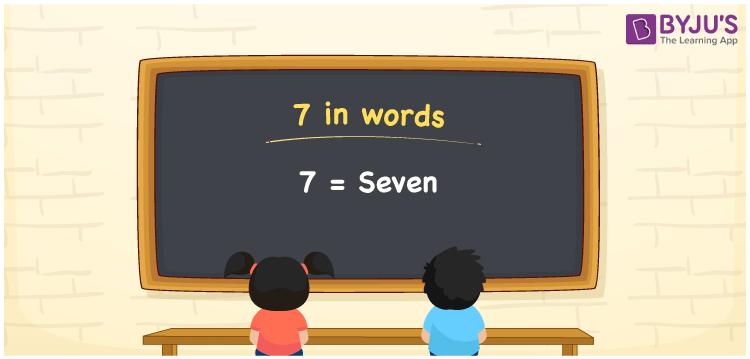# 7 in Words

7 in words is written as Seven. If you gifted 7 toys to a kid, you can say, “I gifted Seven toys to the kid”. In this article, you will learn how to write the number 7 in words, and some interesting facts about 7. Also, note that 7 is a cardinal number since it denotes a certain quantity.

 7 in words Seven Seven in Numbers 7

## 7 in English Words

Generally, we use the English alphabet to write numbers in words using. So, we can read 7 using the words in English as “Seven”.## How to Write 7 in Words?

To write the number 7 in words, we actually do not require a place value chart. As we know, we pronounce counting numbers as one, two, three, and so on. These can be written as:

1 = One

2 = Two

3 = Three

4 = Four

5 = Five

6 = Six

7 = Seven

Therefore, 7 in words = Seven.

7 is a natural number that is the successor of 6 and the predecessor of 8.

7 in words – Seven

Is 7 an even number? – No

Is 7 an odd number? – Yes

Is 7 a composite number? – No

Is 7 a prime number? – Yes

Is 7 a perfect square number? – No

Is 7 a perfect cube number? – No

## Frequently Asked Questions on 7 in Words

Q1

### How do you write 7 in word form?

The spelling of 7 in English is Seven, so the word form of 7 is seven.
Q2

### How do you write Rs. 7 in words?

We can write Rs. 7 in words as Seven rupees.
Q3

### Count the number of letters of the word ADDRESS.

Given word: ADDRESS Number of letters in the word = 7, i.e. seven